# Planetary Gear System

Subjects:

• Sun gear, carrier and ring gear
• Automatic gearbox
• Planetary gear transmissions
• Calculate first gear ratio
• Calculate second gear ratio
• Calculate Third Gear Ratio

Sun gear, carrier and ring gear:
A planetary gear system consists of at least one set of gears, each with a sun gear, a carrier and a ring gear. Basic knowledge about the functioning of the planetary gear system is therefore required (such as turning the sun gear, carrier with satellite wheels and the ring gear, see page automatic gearbox).
Below is an image of a gear set where the sun gear is green, the carrier with the satellite wheels is blue and the ring gear is red. It is clear to see that the gear set is divided in two. The calculation is done with equations, so it doesn't matter if everything is divided by two. After all, the proportions remain the same.

Further on on this page we will calculate with the ratios Z, D and R. By following the lines that connect various planetary systems to each other, the ratios of all Z, D and R can be used to determine the total transmission ratio of the relevant gear. .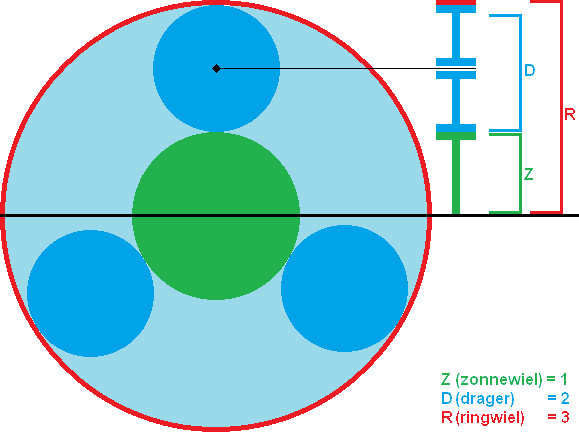Automatic gearbox:
A conventional automatic gearbox works by switching between the different planetary gears, see the chapter automatic gearbox.

Below is a schematic representation of four sets of planetary gear systems in an automatic transmission. There are three systems for the forward gears and one for the reverse. The red line indicates the direction of the forces through the automatic transmission; from the left (engine side with torque converter) through the complete part with planetary systems (black lines) to the propeller shaft coupling. If you look closely at the systems in the gearbox, you will see that the above image is derived from this. Four systems are used in the gearbox, each with a Z, D and R (sun gear, carrier and ring gear).The planetary gear sets are symmetrical above and below the axis. There's no other way, because the interior rotates while driving. To help understand what happens when a gear is engaged, the driven parts in the planetary system shown in the image below have also been made red:In the image above, gear 1 is engaged. To engage gear 1, a clutch must be engaged. This link is shown in blue. With the closed clutch and one driven side of the planetary system, one part must also start to rotate. In that case, the dimensions of the parts determine the transmission ratio (think of a small input gear and a large output gear; the large gear will then rotate more slowly. If the large gear would have twice as many teeth as the small gear, then the ratio would be 1:2).
In principle, this also applies to the automatic transmission; the dimensions of the ring gear, the sun gears and the satellite gears are different in all four systems. Now you can probably imagine that when another clutch is energized (eg from the system on the left) the speed of the output shaft has changed.

Further on this page, images, explanations and calculations make it clear how the planetary gear systems in the automatic gearbox are switched while driving.

Planetary gear train transmissions:
We are now going to look at the top half of the gearbox (because the gearbox is symmetrical above and below, see image below). We will determine the transfers from this image later on the page. Above the systems is the number of the system; from 1 to 3 and system R (backwards).
Each galaxy has its own Z, D, and R. It's not listed in the picture, but if you look again at the picture at the top of this page, you'll recognize it. This will be considered known later on this page.

At the bottom left of the image you see link “K4”, this link ensures that two sides of the system are connected at the same time; system 3 is connected to systems 1 and 2. No other links are closed, so the entire system is “blocked”. The engine speed is transmitted 1 to 1 to the wheels of the vehicle, with no gear ratio involved; we call this price direct. This is in fourth gear.
In cars with a manual gearbox, the fourth gear is often also direct. Here too, the engine speed is transmitted 1 to 1 to the wheels.
The difference in speed of the input shaft (engine or torque converter) and the output shaft (vehicle) is called the gear ratio.First gear is engaged.
By securing the carrier of system I (using coupling K1), a force can be transferred from the sun gear to the carrier. The carrier is connected to the vehicle, so there is now a direct connection between the engine and the gearbox. The dimensions of the parts create a gear ratio (more on this later).

The red line indicates the power curve. The green line indicates which other parts are rotating, because this is directly connected to the red line. These parts turn, but because no clutch is energized, nothing happens to them. They just spin around. The blue line shows what is locked when clutch K1 is energized. Not only the carrier of system 1 is then fixed, but also the carrier of system 3 and the sun gear of system R are blocked.As explained, clutch K1 is energized when shifting into first gear. When shifting to second gear, clutch K1 will be disengaged and another clutch will be energized. That can be seen in the table.

When shifting to second gear, clutch K2 will be energized. The ring gear of system 2 is then fixed. Because the sun gear of system 2 is fixed and the sun gear is driven, the carrier will start to rotate. This carrier will in turn drive system 1. In system 1, this time the ring gear is not blocked, but driven by another system. In that case, the outgoing speed (line of the vehicle) will therefore have a lower speed than when first gear was switched.This is clarified further on this page with images, explanations and calculations.

To calculate the first gear ratio:
According to the table below, link K1 is closed. The ring gear is therefore locked. The drive power from the engine goes through the sun gear and via the carrier to the vehicle. The proportions are also given, namely 1,00 for the sun gear and 3,00 for the ring gear of system 1. We are going to calculate this.
The basic formula for calculating the gear ratios of planetary gear systems is as follows: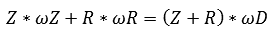ω stands for omega and is the angular velocity while running.

Because we calculate with system 1, we put a 1 after everything. We change this number in the following systems. Certainly in multiple systems (where one system drives the other) it must be noted in this way, because otherwise it will become very cluttered.
Below is the diagram of the first gear. For clarity, the Z (sun gear), D (carrier) and R (ring gear) are drawn in blue.We now fill in the basic formula for the first system. The omegas are unknown and the wearer stands still. So we can't enter anything for this. The Z1 and D1 are known, so we will fill them in. R1 is stationary, so we cross it out. We do not include anything in the formula.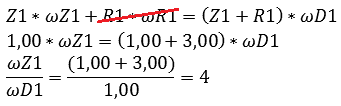You now see that the gear ratio of the first gear is 4.
That never happens in car technology, it would always be slightly above or below 4, because otherwise the gears always touch each other on the same surfaces (extra wear). But here it is easier to calculate as an example. You also now see that the omegas are known!
ωZ1 = 4
ωD1 = 1
These omegas are the angular velocities of the axes in the system. In the first gear, the omegas are not really important yet, but when calculating with double-driven systems (as will become clear in the second gear), it is important.

Calculating second gear ratio:
When calculating the gear ratio of the second gear, it must be taken into account that the first system is double-driven; the sun gear of system 1 is driven by the motor and the carrier is driven by system 2 . A different vehicle speed is now created than in the situation where the ring gear was stationary (as with first gear).When calculating we always start with the system that is only powered. In this case it is therefore system 2, because it is only driven by the motor via the sun gear.The gear the second system performs is 5,1. This is not the transmission between the motor and the wheels, but between the motor and system 1. Now we will calculate the transmission ratio of system 1 with the data from system 2, because the omegas are now known:
ωZ2 = 4,1
ωD2 = 0,8
If you now look in the diagram, you will see that the sun gears of system 1 and 2 are connected to each other. Also, the carrier of system 2 and the ring gear of system 1 are connected to each other. The omegas of the connected parts are the same, so we can then say:
ωZ2 = ωZ1 = 4,1
ωD2 = ωR1 = 0,8
It is very important that this is carefully considered! Always follow the lines in the schedule.

We now enter these omegas in the calculation of system 1.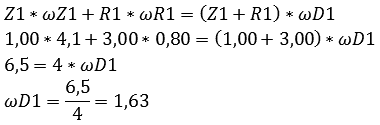We can now determine the final final ratio by dividing the input omega by the outgoing omega. If we look in the diagram we see that the omega of the sun wheel system 2 is ingoing and the omega of the carrier system 1 is outgoing.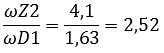So the total gear ratio of the 2nd gear is 2,52.

To calculate the third gear ratio:
When calculating the third gear, it must be taken into account that all three systems work together. Always start with the single drive system. In this case, that's the third:The sun gear of system 3 is fixed, so it does not participate. Then fill in the rest of all values: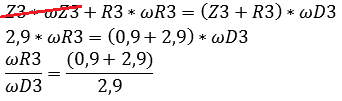With this we obtain: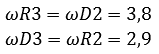Then we go to system 2. The omegas that are known in system 3 are entered in the calculation of system 2: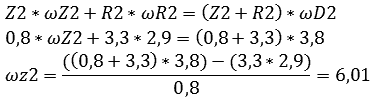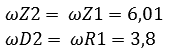Now we go to system 1. The well-known omegas are also filled in here: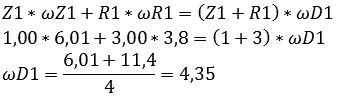In the end we get: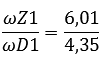That means the total gear ratio of the third gear is 1,38.

To calculate the fourth gear ratio:
Clutch K4 is closed in fourth gear. This means that the sun gears of system 1, 2 and 3 are simultaneously coupled to the motor. The entire system is now blocked. All omegas are equal to each other.If all omegas are equal, no transmission ratio is possible. The engine speed is transmitted directly to the wheels. We call this price direct.error: Alert: Content is protected !!